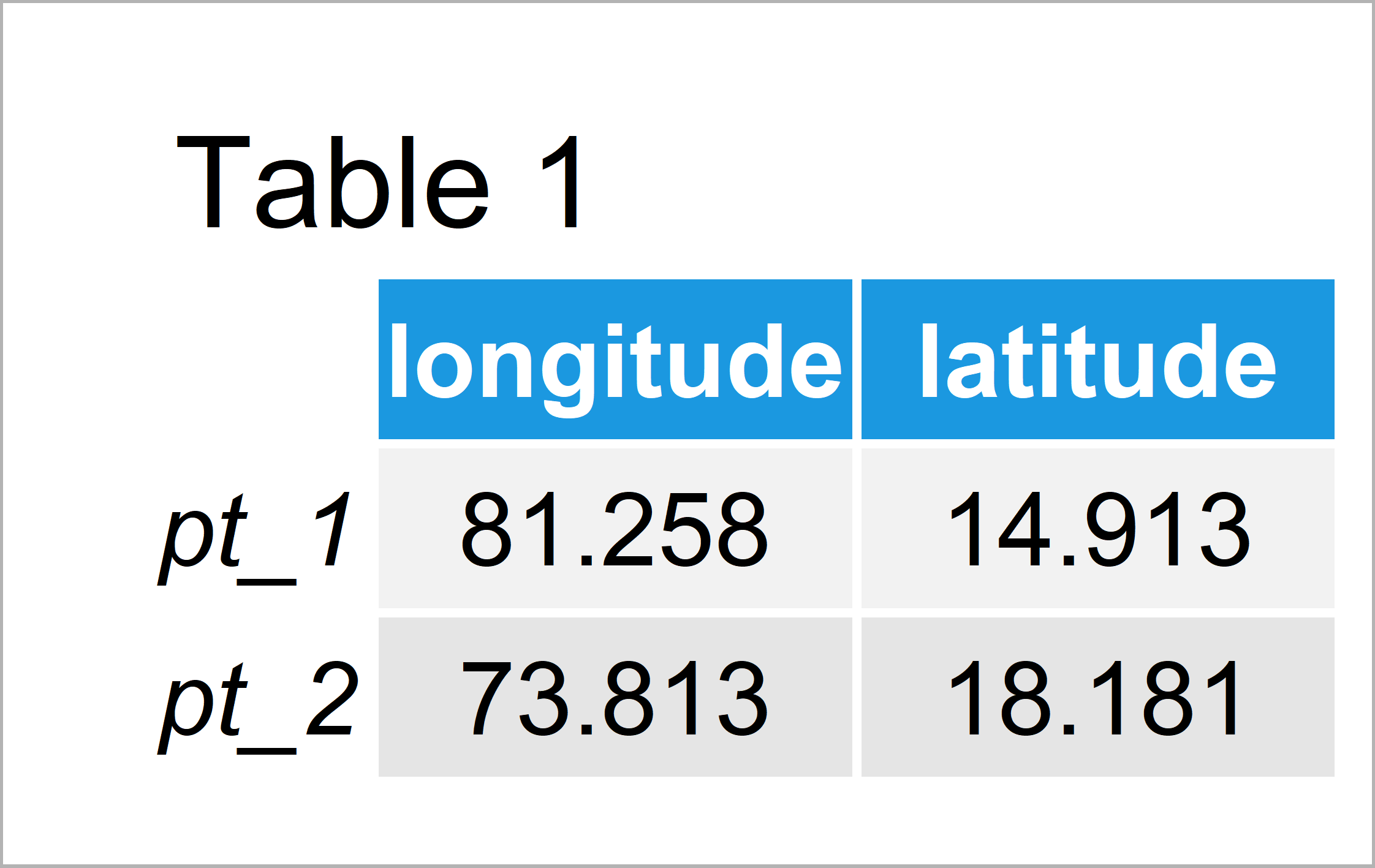# Distance Along Rhumb Line in R (Example)

In this R article you’ll learn how to calculate the distance between two geospatial points along a loxodrome.

Let’s dive right into the exemplifying R code!

## Creation of Example Data

At the start, we’ll have to construct some example data:

```my_points <- matrix(c(81.25798, 73.81277,    # Create longitude/latitude matrix
14.91254, 18.18145),
nrow = 2)
colnames(my_points) <- c("longitude", "latitude")
rownames(my_points) <- c("pt_1", "pt_2")
my_points                                    # Print longitude/latitude matrix```The previous table shows that the example data contains two geographical points stored in two columns.

## Example: Calculate Distance Along a Rhumb Line Using distRhumb() Function of geosphere Package

In this example, I’ll explain how to compute the distance between two geospatial points (i.e. longitude and latitude) along a rhumb line using the distRhumb function.

The distRhumb function is provided by the geosphere package and hence we first have to install and load the geosphere package:

```install.packages("geosphere")                # Install & load geosphere package
library("geosphere")```

As next step, we can apply the distRhumb function:

```my_dist <- distRhumb(my_points)              # Calculate Rhumb distance
my_dist                                      # Print Rhumb distance
#  873730.6```

As you can see based on the previous output of the RStudio console, the distance between our two geospatial points is 873730.6 according to the calculation along a rhumb line.

## Video, Further Resources & Summary

Do you want to know more about geographical distances? Then I recommend having a look at the following video of my YouTube channel. In the video, I explain the R programming codes of this tutorial:

At this point you should have learned how to compute a geospatial distance along a rhumb line in the R programming language. Let me know in the comments section below, in case you have additional questions. Furthermore, don’t forget to subscribe to my email newsletter in order to get updates on the newest articles.

Subscribe to the Statistics Globe Newsletter